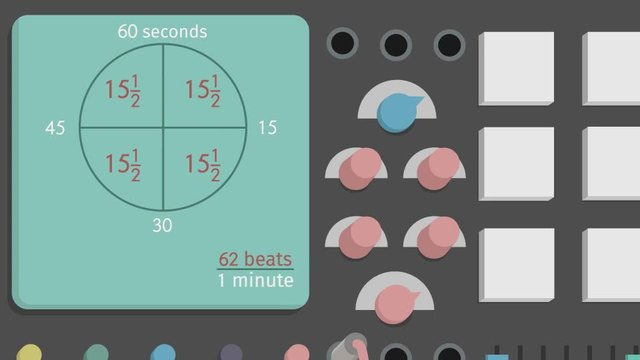# Ratios of FractionsRate this video

Ø 5.0 / 1 ratings

The authorTeam Digital

## Information about the videoRatios of Fractions

After this lesson, you will be able to convert complex fractions to unit rates using visual models to understand a scenario and solve one simple problem.

The lesson begins by teaching you how to use a clock model to find the unit rate involving complex fractions. It leads you to comparing rates. It concludes with using a double number line to compare ratios involving fractions.

Learn how to visualize ratios of fractions by helping Lorenzo and Yoko follow the same beat.

This video includes key concepts, notation, and vocabulary such as complex fractions (fractions with at least one fraction in the numerator or denominator), and visual models (clock models and double number lines) to find unit rates for ratios of complex fractions.

Before watching this video, you should already be familiar with calculating unit rates, interpreting ratios presented in verbal descriptions, ratio tables, graphs, or equations, converting from one representation to another, and assessing whether a relationship represented in any form is proportional or not.

After watching this video, you will be prepared to use division to find the unit rate and compare unit rates involving complex numbers.

Common Core Standard(s) in focus: 7.RP.A.1 A video intended for math students in the 7th grade Recommended for students who are 12 - 13 years old